Probability

# Rearranging Blocks Warmup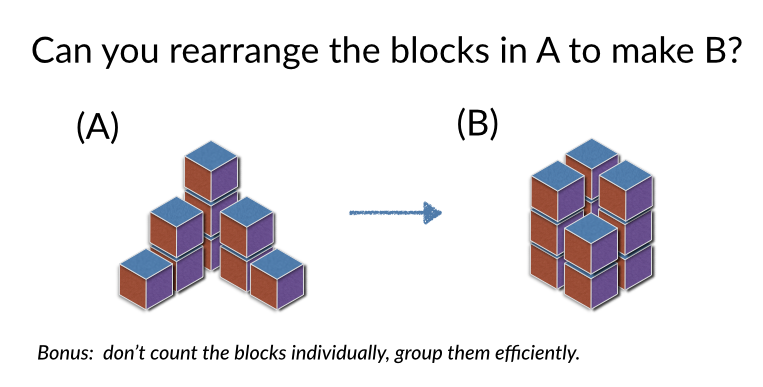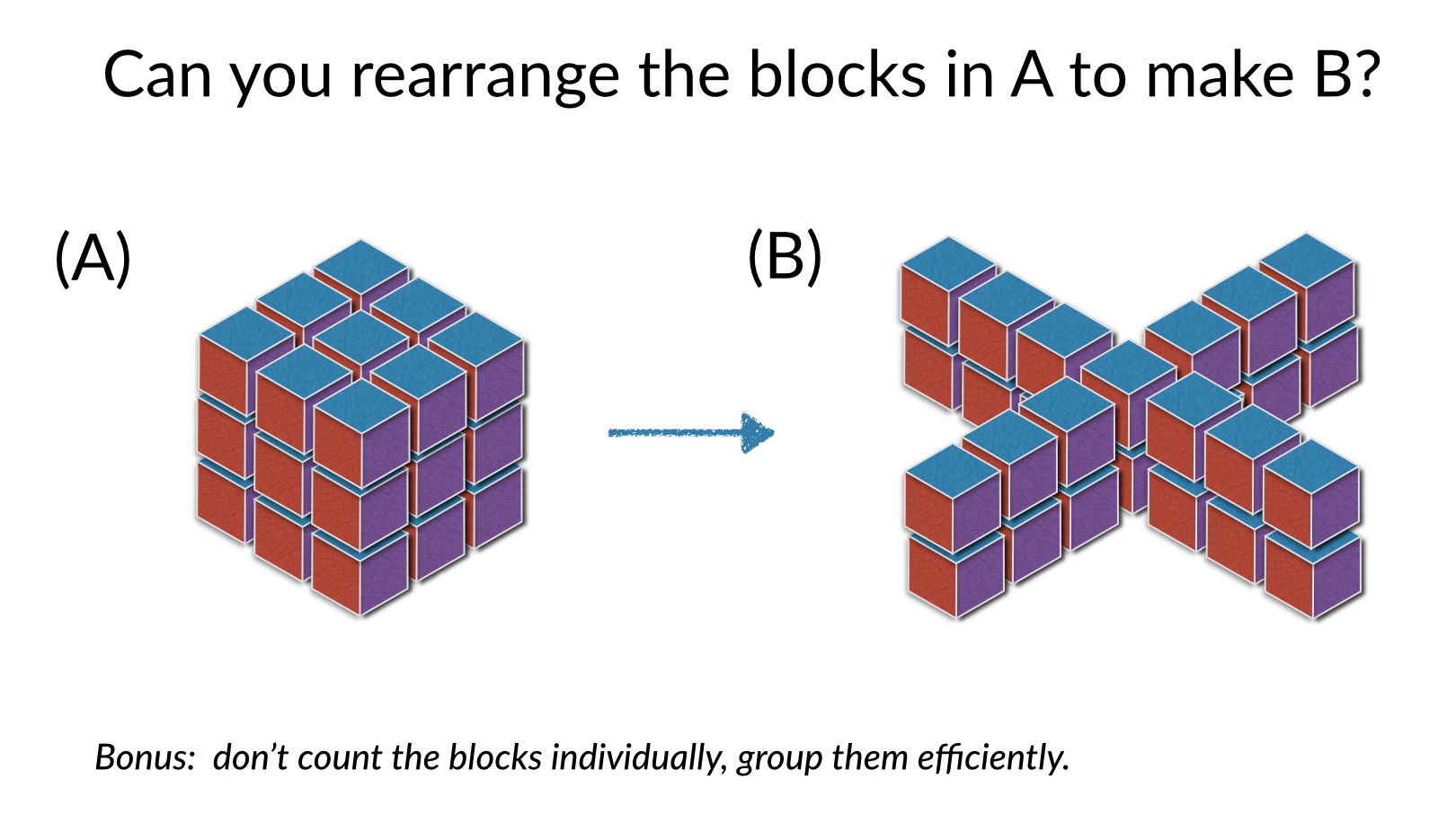(Note: For all questions on this quiz, assume if there is an inner gap where a cube could be, there is a cube. This applies here to the figure on the left.)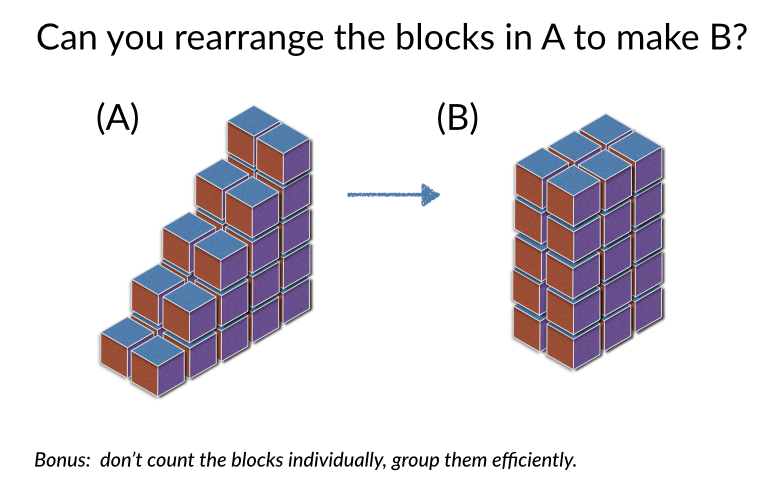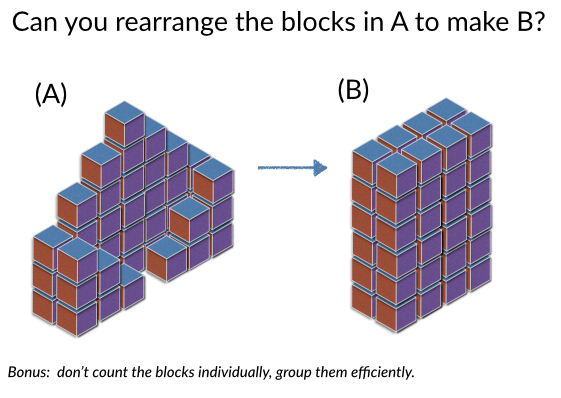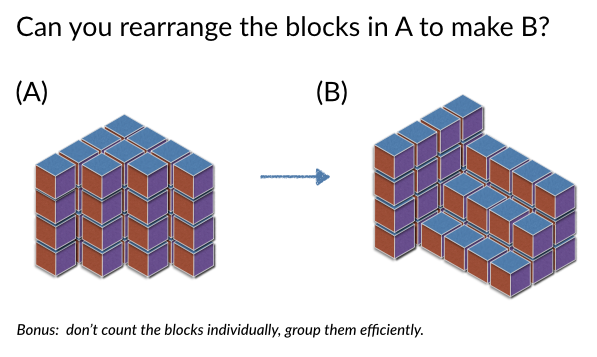×